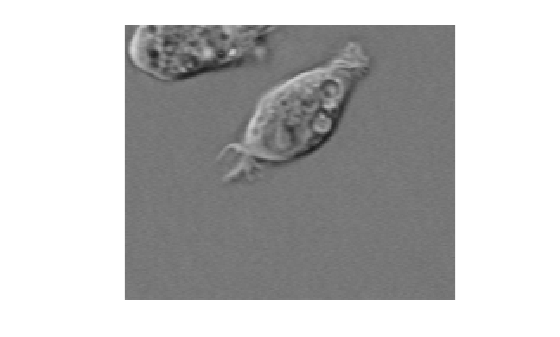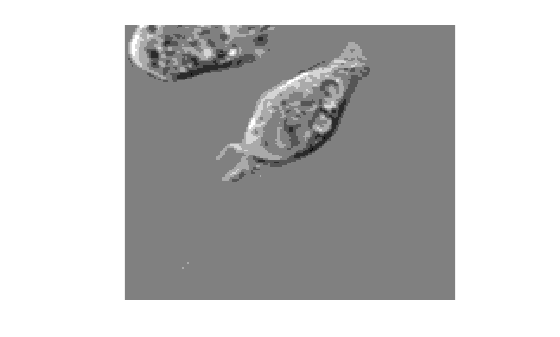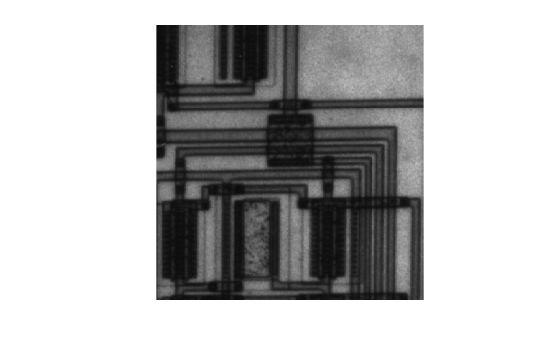# graycomatrix

Create gray-level co-occurrence matrix from image

## Syntax

``glcms = graycomatrix(I)``
``glcms = graycomatrix(I,Name,Value)``
``````[glcms,SI] = graycomatrix(___)``````

## Description

````glcms = graycomatrix(I)` creates a gray-level co-occurrence matrix (GLCM) from image `I`. Another name for a gray-level co-occurrence matrix is a gray-level spatial dependence matrix. `graycomatrix` creates the GLCM by calculating how often a pixel with gray-level (grayscale intensity) value i occurs horizontally adjacent to a pixel with the value j. (You can specify other pixel spatial relationships using the `'Offsets'` parameter.) Each element (i,j) in `glcm` specifies the number of times that the pixel with value i occurred horizontally adjacent to a pixel with value j.```

example

````glcms = graycomatrix(I,Name,Value)` returns one or more gray-level co-occurrence matrices, depending on the values of the optional name-value pair arguments.```

example

``````[glcms,SI] = graycomatrix(___)``` returns the scaled image, `SI`, used to calculate the gray-level co-occurrence matrix.```

## Examples

collapse all

Read a grayscale image into the workspace.

```I = imread('circuit.tif'); imshow(I)```Calculate the gray-level co-occurrence matrix (GLCM) for the grayscale image. By default, `graycomatrix` calculates the GLCM based on horizontal proximity of the pixels: [0 1]. That is the pixel next to the pixel of interest on the same row. This example specifies a different offset: two rows apart on the same column.

`glcm = graycomatrix(I,'Offset',[2 0])`
```glcm = 8×8 14205 2107 126 0 0 0 0 0 2242 14052 3555 400 0 0 0 0 191 3579 7341 1505 37 0 0 0 0 683 1446 7184 1368 0 0 0 0 7 116 1502 10256 1124 0 0 0 0 0 2 1153 1435 0 0 0 0 0 0 0 0 0 0 0 0 0 0 0 0 0 0 ```

Create a simple 3-by-6 sample array.

`I = [ 1 1 5 6 8 8; 2 3 5 7 0 2; 0 2 3 5 6 7]`
```I = 3×6 1 1 5 6 8 8 2 3 5 7 0 2 0 2 3 5 6 7 ```

Calculate the gray-level co-occurrence matrix (GLCM) and return the scaled image used in the calculation. By specifying empty brackets for the `GrayLimits` parameter, the example uses the minimum and maximum grayscale values in the input image as limits.

`[glcm,SI] = graycomatrix(I,'NumLevels',9,'GrayLimits',[])`
```glcm = 9×9 0 0 2 0 0 0 0 0 0 0 1 0 0 0 1 0 0 0 0 0 0 2 0 0 0 0 0 0 0 0 0 0 2 0 0 0 0 0 0 0 0 0 0 0 0 0 0 0 0 0 0 2 1 0 0 0 0 0 0 0 0 1 1 1 0 0 0 0 0 0 0 0 0 0 0 0 0 0 0 0 1 ```
```SI = 3×6 2 2 6 7 9 9 3 4 6 8 1 3 1 3 4 6 7 8 ```

Read a grayscale image into the workspace.

```I = imread('cell.tif'); imshow(I)```Define four offsets.

`offsets = [0 1; -1 1;-1 0;-1 -1];`

Calculate the GLCMs, returning the scaled image as well. Display the scaled image, performing an additional rescaling of data values to the range [0, 1].

```[glcms,SI] = graycomatrix(I,'Offset',offsets); imshow(rescale(SI))```Note how the function returns an array of four GLCMs.

`whos`
``` Name Size Bytes Class Attributes I 159x191 30369 uint8 SI 159x191 242952 double glcms 8x8x4 2048 double offsets 4x2 64 double ```

Read a grayscale image into the workspace.

```I = imread('circuit.tif'); imshow(I)```Calculate the GLCM using the `Symmetric` option, returning the scaled image as well. The GLCM created when you set `Symmetric` to `true` is symmetric across its diagonal, and is equivalent to the GLCM described by Haralick (1973).

```[glcm,SI] = graycomatrix(I,'Offset',[2 0],'Symmetric',true); glcm```
```glcm = 8×8 28410 4349 317 0 0 0 0 0 4349 28104 7134 1083 7 0 0 0 317 7134 14682 2951 153 0 0 0 0 1083 2951 14368 2870 2 0 0 0 7 153 2870 20512 2277 0 0 0 0 0 2 2277 2870 0 0 0 0 0 0 0 0 0 0 0 0 0 0 0 0 0 0 ```

Display the scaled image, performing an additional rescaling of data values to the range [0, 1].

`imshow(rescale(SI))`## Input Arguments

collapse all

Input image, specified as a 2-D numeric matrix or 2-D logical matrix.

### Name-Value Arguments

Specify optional comma-separated pairs of `Name,Value` arguments. `Name` is the argument name and `Value` is the corresponding value. `Name` must appear inside quotes. You can specify several name and value pair arguments in any order as `Name1,Value1,...,NameN,ValueN`.

Example: `'Offset',[2 0]`

Range used for scaling input image into gray levels, specified as a 2-element vector `[low high]`. If `N` is the number of gray levels (see parameter `'NumLevels'`) to use for scaling, the range `[low high]` is divided into `N` equal width bins and values in a bin get mapped to a single gray level. Grayscale values less than or equal to `low` are scaled to 1. Grayscale values greater than or equal to `high` are scaled to `'NumLevels'`. If`'GrayLimits'` is set to `[]`, `graycomatrix` uses the minimum and maximum grayscale values in `I` as limits, ```[min(I(:)) max(I(:))]```, for example, `[0 1]` for class double and `[-32768 32767]` for class `int16`.

Number of gray levels, specified as a positive integer. For example, if `NumLevels` is `8`, then `graycomatrix` scales the values in `I` so they are integers between 1 and 8. The number of gray-levels determines the size of the gray-level co-occurrence matrix (`glcm`). The default number of gray levels is `8` for numeric images and `2` for logical images.

Distance between the pixel of interest and its neighbor, specified as a p-by-2 matrix of integers. Each row in the matrix is a two-element vector, `[row_offset, col_offset]`, that specifies the relationship, or offset, of a pair of pixels. `row_offset` is the number of rows between the pixel-of-interest and its neighbor. `col_offset` is the number of columns between the pixel-of-interest and its neighbor. Because the offset is often expressed as an angle, the following table lists the offset values that specify common angles, given the pixel distance D.

Angle

Offset

0

`[0 D]`

45

`[-D D]`

90`[-D 0]`
135`[-D -D]`

The figure illustrates the array: ```offset = [0 1; -1 1; -1 0; -1 -1]```Consider ordering of values, specified as the Boolean value `true` or `false`. For example, when `'Symmetric'` is set to `true`, `graycomatrix` counts both 1,2 and 2,1 pairings when calculating the number of times the value 1 is adjacent to the value 2. When `'Symmetric'` is set to `false`, `graycomatrix` only counts 1,2 or 2,1, depending on the value of `'offset'`.

Data Types: `logical`

## Output Arguments

collapse all

Gray-level co-occurrence matrix (or matrices), returned as an `NumLevels`-by-`NumLevels`-by-`P` array, where `P` is the number of offsets in `Offset`.

Data Types: `double`

Scaled image used in calculation of GLCM, returned as a numeric matrix of the same size as the input image. The values in `SI` are between `1` and `NumLevels`.

Data Types: `double`

## Algorithms

`graycomatrix` calculates the GLCM from a scaled version of the image. By default, if `I` is a binary image, `graycomatrix` scales the image to two gray-levels. If `I` is an intensity image, `graycomatrix` scales the image to eight gray-levels. You can specify the number of gray levels `graycomatrix` uses to scale the image by using the `'NumLevels'` parameter, and the way that `graycomatrix` scales the values using the `'GrayLimits'` name-value argument.

The following figure shows how `graycomatrix` calculates several values in the GLCM of the 4-by-5 image `I`. Element (1,1) in the GLCM contains the value `1` because there is only one instance in the image where two, horizontally adjacent pixels have the values `1` and `1`. Element `(1,2)` in the GLCM contains the value `2` because there are two instances in the image where two, horizontally adjacent pixels have the values `1` and `2`. `graycomatrix` continues this processing to fill in all the values in the GLCM.`graycomatrix` ignores pixel pairs if either of the pixels contains a `NaN`, replaces positive `Infs` with the value `NumLevels`, and replaces negative `Infs` with the value `1`. `graycomatrix` ignores border pixels, if the corresponding neighbor pixel falls outside the image boundaries.

The GLCM created when `'Symmetric'` is set to `true` is symmetric across its diagonal, and is equivalent to the GLCM described by Haralick (1973). The GLCM produced by the following syntax, with `'Symmetric'` set to `true`

` graycomatrix(I,'offset',[0 1],'Symmetric',true)`

is equivalent to the sum of the two GLCMs produced by the following statements where `'Symmetric'` is set to `false`.

```graycomatrix(I,'offset',[0 1],'Symmetric',false) graycomatrix(I,'offset',[0 -1],'Symmetric',false)```

 Haralick, R.M., K. Shanmugan, and I. Dinstein, "Textural Features for Image Classification", IEEE Transactions on Systems, Man, and Cybernetics, Vol. SMC-3, 1973, pp. 610-621.

 Haralick, R.M., and L.G. Shapiro. Computer and Robot Vision: Vol. 1, Addison-Wesley, 1992, p. 459.